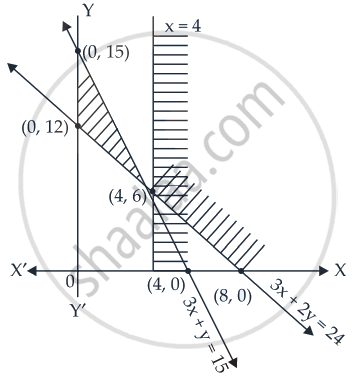# Solve the following system of linear inequalities: 3x + 2y ≥ 24, 3x + y ≤ 15, x ≥ 4 - Mathematics

Graph
Sum

Solve the following system of linear inequalities:

3x + 2y ≥ 24, 3x + y ≤ 15, x ≥ 4

#### Solution

We have, 3x + 2y ≥ 24,

3x +y ≤ 15, x ≥ 4

Now let’s plot lines 3x + 2y = 24, 3x + y = 15 and x = 4 on the coordinate plane.

Line 3x + 2y = 24 passes through the points (0, 12) and (8, 0).

Line 3x+y = 15 passes through points (5, 0) and (0, 15).

Also line x = 4 is passing through the point (4, 0) and vertical.

For (0, 0), 3(0) + 2(0) – 24 < 0.

Therefore, the region satisfying the inequality 3x + 2y ≥ 24 and (0, 0) lie on the opposite of the line 3x + 2y = 24.

For (0), 3(0) + (0) – 15 ≤ 0.

Therefore, the region satisfying the inequality 3x +y ≤ 15 and (0,0) lie on the same side of the line 3x +y = 15.

The region satisfying x ≥ 4 lies to the right-hand side of the line x = 4.

These regions are plotted as shown in the following figure

It is clear from the graph that there is no common region corresponding to these inequalities.Hence, the given system of inequalities has no solution.

Concept: Solution of System of Linear Inequalities in Two Variables
Is there an error in this question or solution?

#### APPEARS IN

NCERT Mathematics Exemplar Class 11
Chapter 6 Linear Inequalities
Exercise | Q 17 | Page 109

Share